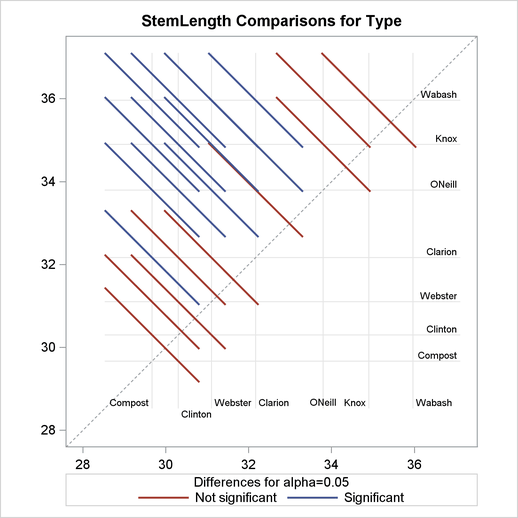LS-Means Diffogram with PROC GLIMMIX

This example is taken from the section Graphics for LS-Mean Comparisons of Chapter 40, The GLIMMIX Procedure. The following statements create a SAS data set that contains measurements from an experiment that investigates how snapdragons grow in various soils:

```data plants;
input Type \$ @;
do Block = 1 to 3;
input StemLength @;
output;
end;
datalines;
Clarion   32.7 32.3 31.5

... more lines ...

;
```

The following statements run PROC GLIMMIX:

```ods graphics on;

proc glimmix data=plants order=data plots=diffogram;
class Block Type;
model StemLength = Block Type;
lsmeans Type;
run;
```

The PLOTS=DIFFOGRAM option produces a diffogram, shown in Figure 21.10, that displays all of the pairwise least squares mean differences and indicates which are significant.

Figure 21.10 LS-Means Diffogram